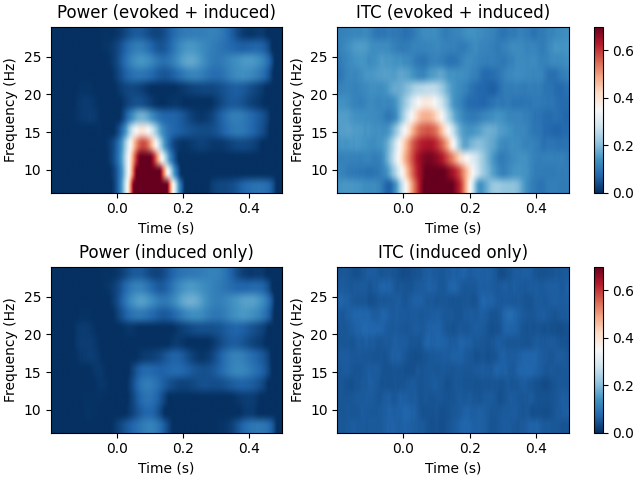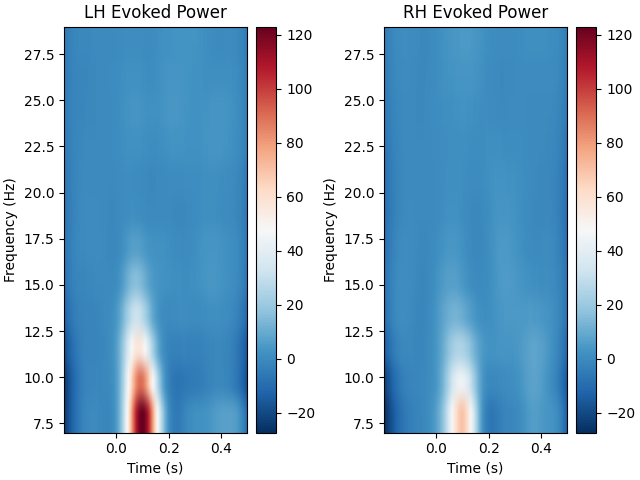# Compute power and phase lock in label of the source space#

Compute time-frequency maps of power and phase lock in the source space. The inverse method is linear based on dSPM inverse operator.

The example also shows the difference in the time-frequency maps when they are computed with and without subtracting the evoked response from each epoch. The former results in induced activity only while the latter also includes evoked (stimulus-locked) activity.

```# Authors: Alexandre Gramfort <alexandre.gramfort@inria.fr>
#
```
```import matplotlib.pyplot as plt
import numpy as np

import mne
from mne import io
from mne.datasets import sample

print(__doc__)
```

Set parameters

```data_path = sample.data_path()
meg_path = data_path / "MEG" / "sample"
raw_fname = meg_path / "sample_audvis_raw.fif"
fname_inv = meg_path / "sample_audvis-meg-oct-6-meg-inv.fif"
label_names = ["Aud-lh", "Aud-rh"]
fname_labels = [meg_path / "labels" / f"{ln}.label" for ln in label_names]

tmin, tmax, event_id = -0.2, 0.5, 2

# Setup for reading the raw data
events = mne.find_events(raw, stim_channel="STI 014")

include = []

# Picks MEG channels
picks = mne.pick_types(
raw.info, meg=True, eeg=False, eog=True, stim=False, include=include, exclude="bads"
)

epochs = mne.Epochs(
raw,
events,
event_id,
tmin,
tmax,
picks=picks,
baseline=(None, 0),
reject=reject,
)

# Compute a source estimate per frequency band including and excluding the
# evoked response
freqs = np.arange(7, 30, 2)  # define frequencies of interest
labels = [mne.read_label(fl) for fl in fname_labels]
label = labels
n_cycles = freqs / 3.0  # different number of cycle per frequency

# subtract the evoked response in order to exclude evoked activity
epochs_induced = epochs.copy().subtract_evoked()

fig, axes = plt.subplots(2, 2, layout="constrained")
for ii, (this_epochs, title) in enumerate(
zip([epochs, epochs_induced], ["evoked + induced", "induced only"])
):
# compute the source space power and the inter-trial coherence
power, itc = source_induced_power(
this_epochs,
inverse_operator,
freqs,
label,
baseline=(-0.1, 0),
baseline_mode="percent",
n_cycles=n_cycles,
n_jobs=None,
)

power = np.mean(power, axis=0)  # average over sources
itc = np.mean(itc, axis=0)  # average over sources
times = epochs.times

##########################################################################
# View time-frequency plots
ax = axes[ii, 0]
ax.imshow(
20 * power,
extent=[times, times[-1], freqs, freqs[-1]],
aspect="auto",
origin="lower",
vmin=0.0,
vmax=30.0,
cmap="RdBu_r",
)
ax.set(xlabel="Time (s)", ylabel="Frequency (Hz)", title=f"Power ({title})")

ax = axes[ii, 1]
ax.imshow(
itc,
extent=[times, times[-1], freqs, freqs[-1]],
aspect="auto",
origin="lower",
vmin=0,
vmax=0.7,
cmap="RdBu_r",
)
ax.set(xlabel="Time (s)", ylabel="Frequency (Hz)", title=f"ITC ({title})")
fig.colorbar(ax.images, ax=axes[ii])
``````Opening raw data file /home/circleci/mne_data/MNE-sample-data/MEG/sample/sample_audvis_raw.fif...
Read a total of 3 projection items:
PCA-v1 (1 x 102)  idle
PCA-v2 (1 x 102)  idle
PCA-v3 (1 x 102)  idle
Range : 25800 ... 192599 =     42.956 ...   320.670 secs
320 events found on stim channel STI 014
Event IDs: [ 1  2  3  4  5 32]
Reading inverse operator decomposition from /home/circleci/mne_data/MNE-sample-data/MEG/sample/sample_audvis-meg-oct-6-meg-inv.fif...
[done]
[done]
305 x 305 full covariance (kind = 1) found.
Read a total of 4 projection items:
PCA-v1 (1 x 102) active
PCA-v2 (1 x 102) active
PCA-v3 (1 x 102) active
Average EEG reference (1 x 60) active
22494 x 22494 diagonal covariance (kind = 2) found.
22494 x 22494 diagonal covariance (kind = 6) found.
22494 x 22494 diagonal covariance (kind = 5) found.
Did not find the desired covariance matrix (kind = 3)
Computing patch statistics...
[done]
Computing patch statistics...
[done]
Read a total of 4 projection items:
PCA-v1 (1 x 102) active
PCA-v2 (1 x 102) active
PCA-v3 (1 x 102) active
Average EEG reference (1 x 60) active
Source spaces transformed to the inverse solution coordinate frame
73 matching events found
Setting baseline interval to [-0.19979521315838786, 0.0] s
Applying baseline correction (mode: mean)
Created an SSP operator (subspace dimension = 3)
3 projection items activated
Rejecting  epoch based on EOG : ['EOG 061']
Rejecting  epoch based on EOG : ['EOG 061']
Rejecting  epoch based on EOG : ['EOG 061']
Rejecting  epoch based on EOG : ['EOG 061']
Rejecting  epoch based on EOG : ['EOG 061']
Rejecting  epoch based on MAG : ['MEG 1711']
Rejecting  epoch based on EOG : ['EOG 061']
Rejecting  epoch based on EOG : ['EOG 061']
Rejecting  epoch based on EOG : ['EOG 061']
Rejecting  epoch based on EOG : ['EOG 061']
Rejecting  epoch based on EOG : ['EOG 061']
Rejecting  epoch based on EOG : ['EOG 061']
Rejecting  epoch based on EOG : ['EOG 061']
Rejecting  epoch based on EOG : ['EOG 061']
Subtracting Evoked from Epochs
The following channels are not included in the subtraction: EOG 061
[done]
Preparing the inverse operator for use...
Scaled noise and source covariance from nave = 1 to nave = 1
Created the regularized inverter
Created an SSP operator (subspace dimension = 3)
Created the whitener using a noise covariance matrix with rank 302 (3 small eigenvalues omitted)
Computing noise-normalization factors (dSPM)...
[done]
Picked 305 channels from the data
Computing inverse...
Eigenleads need to be weighted ...
Reducing data rank 99 -> 99
Computing source power ...
Outputting power for 33 vertices in label Aud-lh.
Applying baseline correction (mode: percent)
Preparing the inverse operator for use...
Scaled noise and source covariance from nave = 1 to nave = 1
Created the regularized inverter
Created an SSP operator (subspace dimension = 3)
Created the whitener using a noise covariance matrix with rank 302 (3 small eigenvalues omitted)
Computing noise-normalization factors (dSPM)...
[done]
Picked 305 channels from the data
Computing inverse...
Eigenleads need to be weighted ...
Reducing data rank 99 -> 99
Computing source power ...
Outputting power for 33 vertices in label Aud-lh.
Applying baseline correction (mode: percent)
```

In the example above, we averaged power across vertices after calculating power because we provided a single label for power calculation and therefore power of all sources within the single label were returned separately. When we provide a list of labels, power is averaged across sources within each label automatically. With a list of labels, averaging is performed before rescaling, so choose a baseline method appropriately.

```# Get power from multiple labels
multi_label_power = source_induced_power(
epochs,
inverse_operator,
freqs,
labels,
baseline=(-0.1, 0),
baseline_mode="mean",
n_cycles=n_cycles,
n_jobs=None,
return_plv=False,
)

# visually compare evoked power in left and right auditory regions
fig, axes = plt.subplots(ncols=2, layout="constrained")
for l_idx, l_power in enumerate(multi_label_power):
ax = axes[l_idx]
ax.imshow(
l_power,
extent=[epochs.times, epochs.times[-1], freqs, freqs[-1]],
aspect="auto",
origin="lower",
vmin=multi_label_power.min(),
vmax=multi_label_power.max(),
cmap="RdBu_r",
)
title = f"{labels[l_idx].hemi.upper()} Evoked Power"
ax.set(xlabel="Time (s)", ylabel="Frequency (Hz)", title=title)
fig.colorbar(ax.images, ax=ax)
``````Preparing the inverse operator for use...
Scaled noise and source covariance from nave = 1 to nave = 1
Created the regularized inverter
Created an SSP operator (subspace dimension = 3)
Created the whitener using a noise covariance matrix with rank 302 (3 small eigenvalues omitted)
Computing noise-normalization factors (dSPM)...
[done]
Picked 305 channels from the data
Computing inverse...
Eigenleads need to be weighted ...
Reducing data rank 180 -> 180
Computing source power ...
Averaging induced power across vertices within labels for 2 labels.
Applying baseline correction (mode: mean)
```

Total running time of the script: (0 minutes 20.744 seconds)

Estimated memory usage: 230 MB

Gallery generated by Sphinx-Gallery